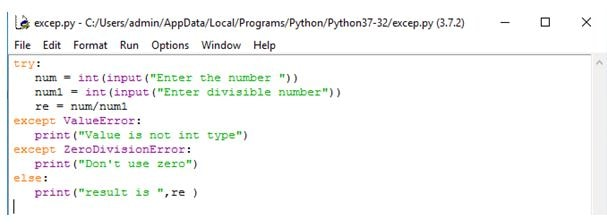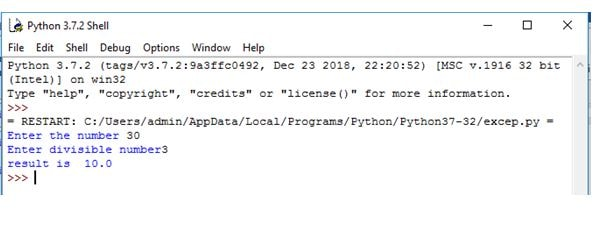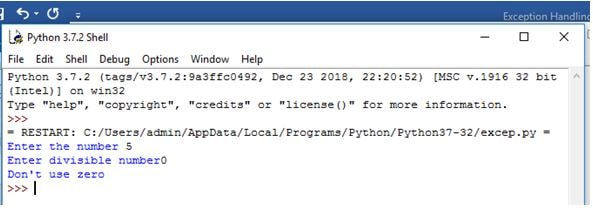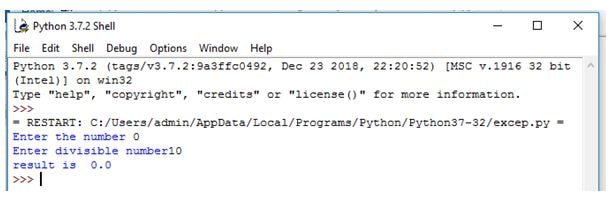Don't let the Lockdown slow you Down - Enroll Now and Get 3 Course at 24,999/- Only. Explore Now!

# General# Python Exception Handling

## Exception Handling

• Exception handling is used to handle the errors which can be try to catch it, like zero divisible by any number.
• Error is nothing but shows the syntax error in any language.

### Method 1:

#### Syntax

```try
operation block;
except Exception_name:
If there is Exception_name1 then execute this block.
except Exception_name2:
If there is Exception_name2, then execute this block.
else
If there is no exception then execute this block.```

#### Example:

```try:
num = int(input("Enter the number "))
num1 = int(input("Enter divisible number"))
re = num/num1
except ValueError:
print("Value is not int type")
except ZeroDivisionError:
print("Don't use zero")
else:
print("result is ",re )
```

Step 1:Step 2:Step 3:Step 4:Step 5:### Method 2:

#### Syntax

```try:
#run this action first
except:
# Run if exception occurs
Finally :
#Always run this code```

#### Example

```try:
num = int(raw_input("Enter the number "))
re = 100/num
except:
print "Something is wrong"
else:
print "result is ",re
finally :
print "finally program ends"```